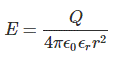# Electric Flux and Electric Flux Density

Electric flux is defined as the total number of electric lines of force emanating from a charged body. An electric field is represented by electric flux.

Like magnetic flux, electric flux lines are not always closed loop. This is because, an isolated magnetic north pole or an isolated magnetic south pole do not exist practically, but an isolated positively charged body and an isolated negatively charged body can exist. We generally denote electric flux with Ψ. We take the measuring unit of flux as the amount flux emanated from one coulomb positive electric charge. We know that number of lines of force emanated from a positive charge body is numerically equal to the charge of the body measured in coulomb. As the flux is total number of lines of force emanated from the charge body, the unit of flux is also taken as Coulomb. So, if Q is the charge of a body and Ψ is the electric flux emanated from the body, then we can write,
Q = Ψ

## Electric Flux Density

Electric flux density is defined as the amount of flux passes through unit surface area in the space imagined at right angle to the direction of electric field. The expression of electric field at a point is given byWhere, Q is the charge of the body by which the field is created. R is the distance of the point from the center of the charged body.
As, we know, Q = ΨThe above equation can be rewritten as,This is the expression of flux per unit area since, 4πr2 is the surface area of the imaginary spare of radius r. This is the flux passing through per unit area at a distance r from the center of the charge. This is called electric flux density at the said point. We generally denote it with English letter D. Therefore,From, the above expression of D, it is clear that electric field intensity and electric field density are in same phasor.

As the number of electric lines of force emanated from a charged body is equal to the quantity of charge of the body measured in coulombs, we can also define the electric flux density at any point in the electric field of the body as the number of lines of force passing through a unit surface area at that point.

Want To Learn Faster? 🎓
Get electrical articles delivered to your inbox every week.
No credit card required—it’s 100% free.